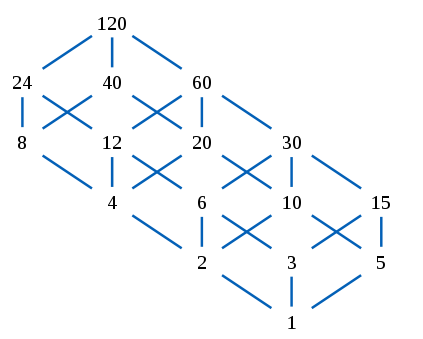# Divisible Sequences

Probability Level 4We want to create a divisible sequence of length $l$ starting from a number $N$. In a Divisible Sequence, every term (except the starting number) is a divisor of the previous term. Examples of divisible sequences of length 3 starting with 10 are:

$10,10,10$

$10,10,5$

$10,10,1$

$10,5,5$ ... etc.

Find the number of divisible sequences of length 5 starting from the number 360.

×

Problem Loading...

Note Loading...

Set Loading...